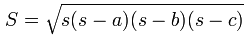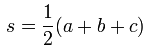Heron's formula Calculation Program

Information
• You can input Integer, fraction, and decimal. Values must be larger than 0.
• Number of characters that can be input, is 7.
• About fraction input:
• For ex, "2/3"(two thirds) - please input "2" first, and "/", "3".
• In case of mixed number fraction - for ex "3+4/5".
• At each processing, an internal calculation uses the value in which the sixth place of the decimal point is rounded up when cannot it be done after it converts it into the decimal, and it be divided even if it is an input by the fraction.

Heron's formula The area of a triangle(S) with sides of length a, b, c is :whereCalculate

Please input lengths of 3 sides, and push "Run"